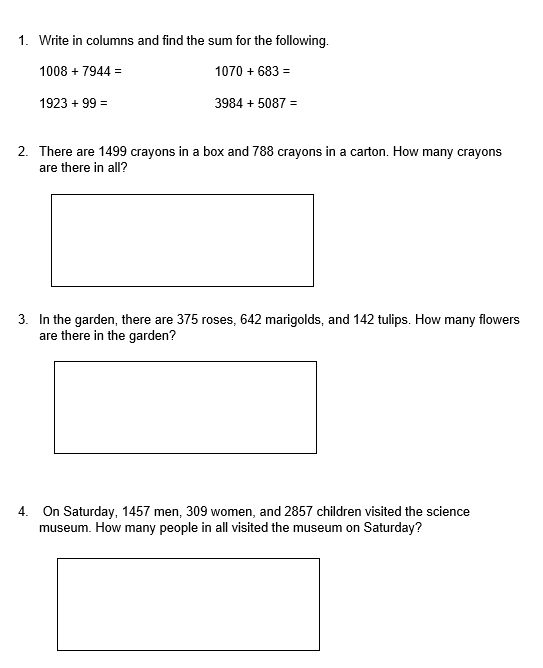# Class 3 Math Chapter 2 Worksheet (PDF)

This worksheet contains counting numbers higher than 100 and is the subject of the chapter Fun with Numbers. It also includes forward and reverses counting for numbers greater than 100. Word problems are used to further explain the idea of addition and subtraction.

## What is the “Class 3 Math Chapter 2 Worksheet (PDF)”?

This worksheet Class 3 Math Chapter 2 Worksheet (PDF). It elaborates on the concept of addition and subtraction through word problems. Also, to add three digits and how to regroup while adding.

How will the “Class 3 Math Chapter 2 Worksheet (PDF)” help you?

Instructions on how to use the “Class 3 Math Chapter 2 Worksheet (PDF).”

Examine the theory and examples provided, then attempt to complete the exercises below.

## Conclusion

This worksheet can ease your problem in tutoring your kids about numbers. This worksheet provided complete details about numbers such as numbers into words and how to regroup while adding.

If you have any inquiries or feedback, please let us know.

## Class 3 Math Chapter 2 Worksheet (PDF)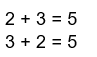1. Numbers can be added in any order.
1.  When 1 is added to a number,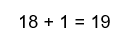The sum is the number after.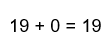1. When 0 is added to a number,

The sum is the number itself.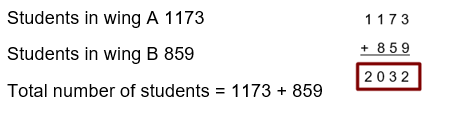1. Worksheet